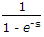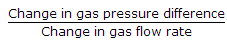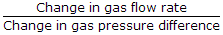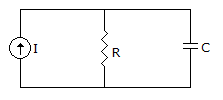# Electronics and Communication Engineering - Automatic Control Systems

### Exercise :: Automatic Control Systems - Section 6

21.

For the function G(s) =the poles are at

 A. s = 0 and s = ∞ B. s = j2np where n = 0, 1, 2 C. s = ± j2np where n = 0, 1, 2.. D. none of the above.

Explanation:

No answer description available for this question. Let us discuss.

22.

Which dc servomotor has minimum inertia?

 A. Slotted armature construction B. Surface wound armature C. Surface wound with stationary rotor D. Either (a) or (b)

Explanation:

No answer description available for this question. Let us discuss.

23.

Gas flow resistance is defined as

 A.B.C. (change in gas pressure difference) x (change in gas flow rate) D. none of the above

Explanation:

No answer description available for this question. Let us discuss.

24.

Examine the following statements

1. A small size hydraulic actuator can develop good torque.
2. It is easy to maintain a hydraulic system leak proof.
3. Both linear and rotatary hydraulic actuators are available.
4. Hydraulic power is more easily available than electric power.
Which of the above statements are correct?

 A. all B. 1 and 2 only C. 1 and 3 only D. 2, 3 and 4 only

Explanation:

No answer description available for this question. Let us discuss.

25.

The given figure shows a constant current source driving a parallel RC circuit. It is equivalent toA. a lag network B. a lead network C. a lag-lead network D. either (a) or (b)